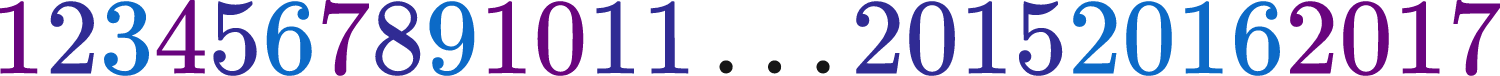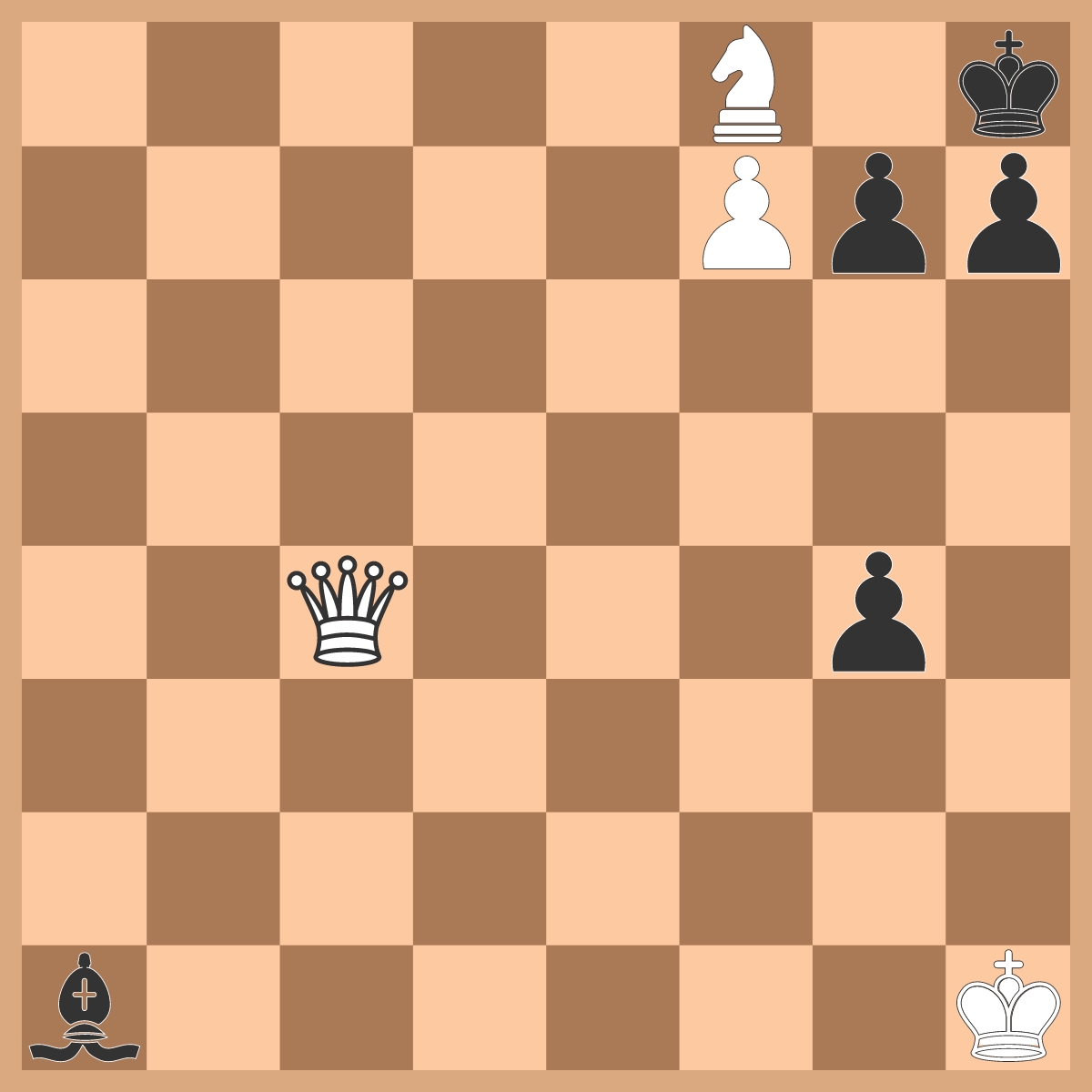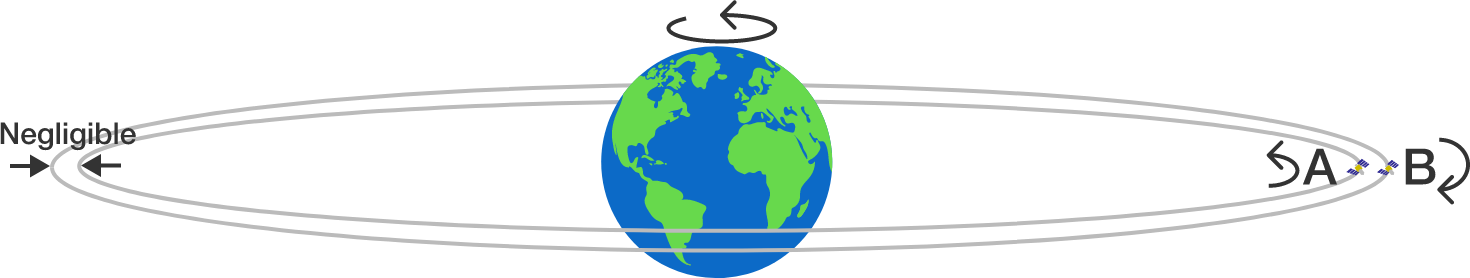# Problems of the Week

Contribute a problem

# 2017-05-08 Intermediate

How many digits does the following number have?It’s White's turn to move. If both White and Black play optimally, what is the minimum number of moves for White to checkmate the Black king?

True or False?

If a complex number $N$ satisfies $N (N + 1)(N + 2)=5 \times 6 \times 7,$ then it must be the case that $(N+6)(N+7)(N+8) =11\times12\times13.$Two identical satellites $A$ and $B$ are launched in equatorial circular orbits around Earth with the same period of revolution of 4.8 hours. Satellite $A$ rotates west to east (with Earth's rotation) while satellite $B$ rotates east to west (against Earth's rotation).

On a particular day, both satellites were seen directly overhead by an observer standing at point $P$ on Earth’s equator. How long (in hours) will it be until both satellites are seen directly overhead again from the same point $P?$


Details and Assumptions:

• The orbits are located on nearly the same circle, with just enough separation to avoid collision.

$\dfrac{a-b}{c-d} = 2, \qquad \dfrac{a-c}{b-d} = 3$

If the above are true for real numbers $a$, $b$, $c$, and $d$, find the value of $\dfrac{a-d}{b-c}.$

×